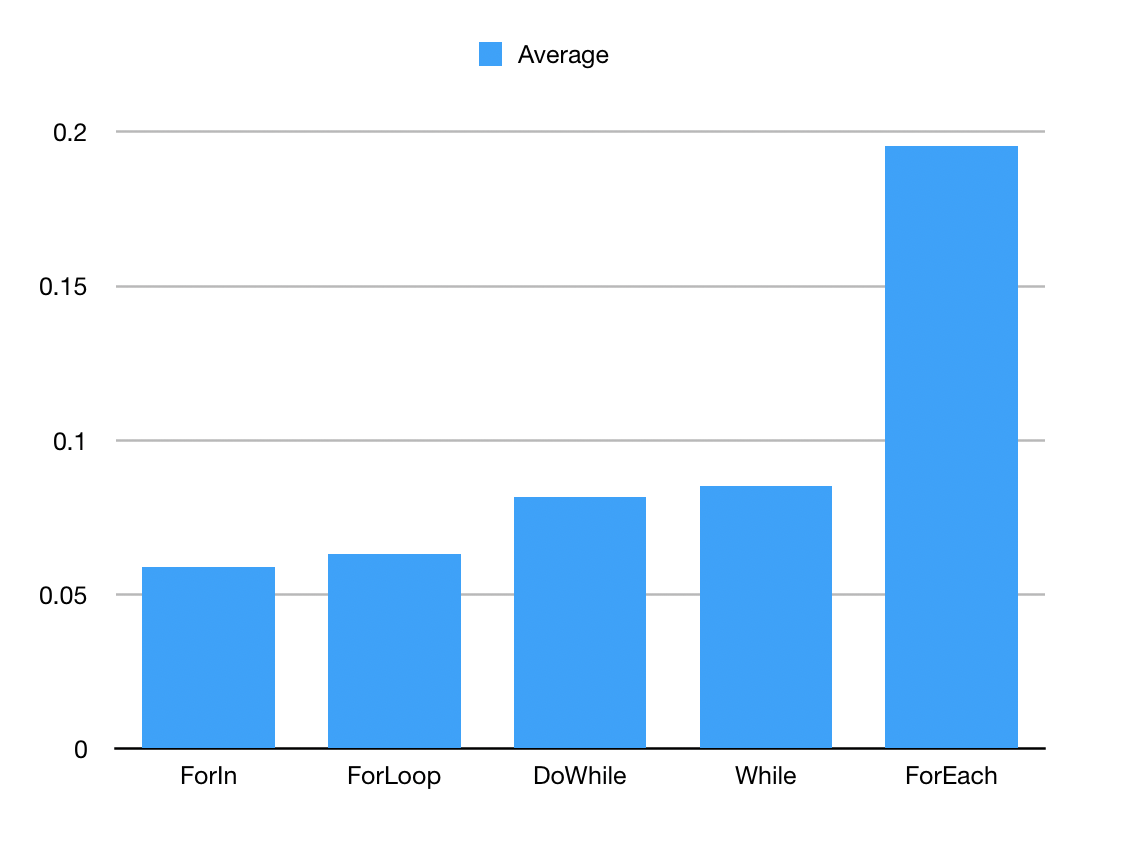There are a few different ways to do loops in JavaScript. Lets take a look at which one is the fastest.

## Setup

I’m running this on my Mac, with a 2 GHz Intel Core i5 with Node v12.1.0. Lets have an array that we just run through and do some basic operations.

Basic setup of the array

``````var arr = new Array(1000)
for(var i = 0; i < arr.length; i++){
arr[i] = i
}
``````

#### For Loop

``````var sum = 0
for(var i = 0; i < arr.length; i++){
sum += arr[i] * 2
}
console.log("SUM: " + sum);
``````

#### Do While Loop

``````var sum = 0
var i = 0
do {
sum += arr[i] * 2
i++
} while ( i < arr.length )
console.log("SUM: " + sum);
``````

#### While Loop

``````var sum = 0
var i = 0
while (i < arr.length) {
sum += arr[i] * 2
i++
}
console.log("SUM: " + sum);
``````

#### For Of

``````var sum = 0
for(var i of arr){
sum += i * 2
}
console.log("SUM: " + sum);
``````

#### For Each

``````var sum = 0
arr.forEach(i => {
sum += i * 2
})

console.log("SUM: " + sum);
``````

You can take a look at all the code on Github.

## The Test

So each loop got its own file and a simple Ruby program timed each one. After ten thousand times hopefully a pattern emerges. Its entirely possible that all the loops run in the same amount of time since this is all being converted to a lower level.

## ResultsLoop Average (s)
For Of 0.0590751585999955
For Loop 0.0632383086000213
Do While 0.0814892920999774
While 0.08505968210001450
For Each 0.195300551599986

There you have it. For-in is the fastest but is not recommended since it can be out of order, leaving the For loop as the best/fastest loop. That being said for each is an easy option but terribly slow in comparison.